# ISEE Middle Level Math : Data Analysis

## Example Questions

### Example Question #71 : Data Analysis

Use the following data set to answer the question.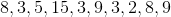Find the range of the data set.

Possible Answers:Correct answer:Explanation:

To find the range of a data set, we will find the difference of the smallest and the largest number within the set.  So, in the setwe can see the smallest number is 2 and the largest number is 15.  To find the difference of these two numbers, we will subtract them.  So,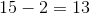Therefore, the range of the data set is.

### Example Question #70 : Range

What is the range of the following data set?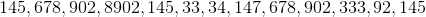Possible Answers: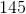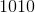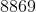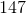Correct answer:Explanation:

What is the range of the following data set?To find the range of a data set, simply find the difference between the least and greatest values.

Here, our largest value is 8902

Our least value is 33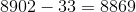So our answer is 8069

### Example Question #72 : Data Analysis

Use the following data set to answer the question.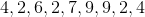Find the range.

Possible Answers:Correct answer:Explanation:

To find the range of a data set, we will take the smallest and largest number and calculate the difference of those two numbers.

So, given the data setwe can see the smallest number isand the largest number is.  Now, we will find the difference.  We get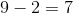Therefore, the range of the data set is.

### Example Question #72 : Data Analysis

Find the range of the following data set: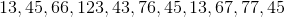Possible Answers: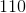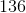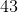Correct answer:Explanation:

Find the range of the following data set:Let's begin by ordering our data set from least to greatest.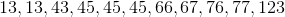To find the range, we need to find the difference between the greatest and least values.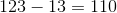So our answer is 110

### Example Question #73 : Data Analysis And Probability

Use the following data set to answer the question.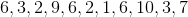Find the range.

Possible Answers:Correct answer:Explanation:

To find the range of a data set, we must first find the smallest number and the largest number within the set.  Once we do that, we must find the difference of those numbers, or subtract those two numbers.

So, given the setwe can see the smallest number isand the largest number is. Now, we will find the difference.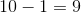Therefore, the range of the data set is.

### Example Question #71 : Data Analysis And Probability

If the middle number of a set is increased, how is the range affected?

Possible Answers:

Cannot be determined

Increases

Decreases

No effect

Correct answer:

Cannot be determined

Explanation:

The reason that this cannot be determined is that there is no specific increase given in the problem.  The minimum number and maximum number are used to find the range.  If the middle number remains below the maximum number, the range remains the same.  If the original middle number is increased to the point of being larger than the original maximum, the range will increase.

### Example Question #75 : Data Analysis And Probability

Find the range of the following data set: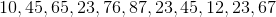Possible Answers: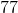Correct answer:Explanation:

Find the range of the following data set:First, let's reorder our data set: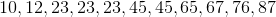Now, simply find the difference between our largest and smallest numbers: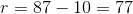So our answer is 77

### Example Question #75 : Data Analysis And Probability

Use the following data set to answer the question.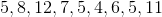Find the range of the data set.

Possible Answers:Correct answer:Explanation:

To find the range of a data set, we will first locate the smallest and the largest numbers within the set.  Once we do that, we will then find the difference of those two numbers.  To find the difference, we simply subtract the numbers.

So, given the setwe can see the smallest number isand the largest number is.  Now, we will find the difference.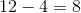Therefore, the range of the data set is.

### Example Question #76 : Data Analysis And Probability

Use the following data set to answer the question.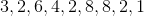Find the range of the data set.

Possible Answers:Correct answer:Explanation:

To find the range of a data set, we will first locate the smallest and the largest numbers within the set.  Once we do that, we will then find the difference of those two numbers.  To find the difference, we simply subtract the numbers.

So, given the setwe can see the smallest number isand the largest number is.  Now, we will find the difference.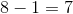Therefore, the range of the data set is.

### Example Question #71 : Data Analysis And Probability

Find the range of the following data set.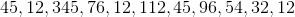Possible Answers: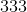Correct answer:Explanation:

Find the range of the following data set.To begin, put the terms in ascending order: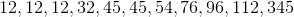Next, find the difference between the first and last numbers: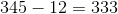So, our range is 333

### All ISEE Middle Level Math Resources